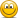Regulation CRM

kunduanil

New Member
Hi David,

In core readings under CRM is mentioned under standardized approach but in you have mentioned it after explaining both standardized approach and IRB.does that mean Comprehensive Approach (to CRM):
will be same under both categories.

Anil

David Harper CFA FRM

David Harper CFA FRM
Staff member
Subscriber
Hi Anil,

No. Simple and comprehensive are standardized approaches (they modify the "basic" basel approach which is to multiply an exposure by a risk weight).
But IRB is the "advanced" approach (based on the same principles we study in credit risk), EL = PD*EAD*LGD. So, the CRM IRB needs a different approach anyhow.
Here is my blog entry on basel ii CRM that should help.

1. standardized (two sub-approaches: simple and comprehensive)
2. IRB.

This Monday's episode includes Basel II, starting with an overview.

David

kunduanil

New Member
Hi David,

didnt get why are we doing this in your blog:

the risk weight of the collateral is substituted for the risk weight of the counterparty under simple approach.

i mean we need to adjust riskweight but why subsititing riskweight of collateral with counterparty.i mean it should be counterparty but what does the risk weight of collateral implies over here.

is it related to correlation between counterparty and the collateral.like when PD of default increases collateral value decreases.so we are using risk weight of counterparty instead of risk weight of collateral which is constant(which i dont know should be constant or not).

Anil

David Harper CFA FRM

David Harper CFA FRM
Staff member
Subscriber
Hi Anil,

Under simple:

If the loan is unsecured, then *simple* Basel II charge is:
Loan Amount * risk weight * 8% (i.e., same Cooke ratio as current Basel; i.e., hold equity against 8% )

If loan is (validly) collateralized, then bank can replace the risk weight above with the risk weight for the collateral. So maybe the risk weight above was 100% (corporate junk bond weight) and, thusly, the capital against the unsecured loan is 8%. But instead the collateral is cash. Then the risk weight is 20%/0%. Now the charge is:
Loan Amount * [risk weight for cash = 20%/0%] * 8%

So it's just a substitution of risk weight, for the collateralized portion of course, and the charge will be lowerered if the collateral is "higher quality" (lower weight)

Then the comprehensive is different than this, it adjusts the exposure instead of the risk weight. To your original question, both simple and comprehensive are acting on:

risk weight * exposure, but
simple alters (substitutes) the risk weight, and
comprehensive (after netting the collateral) alters (haircuts) the exposure

And, to your original question, the IRB acts on the LGD. Arguably the most intuitive of all! Collateral ought to reduce the loss given default.

David

kunduanil

New Member
thanks david..that helps